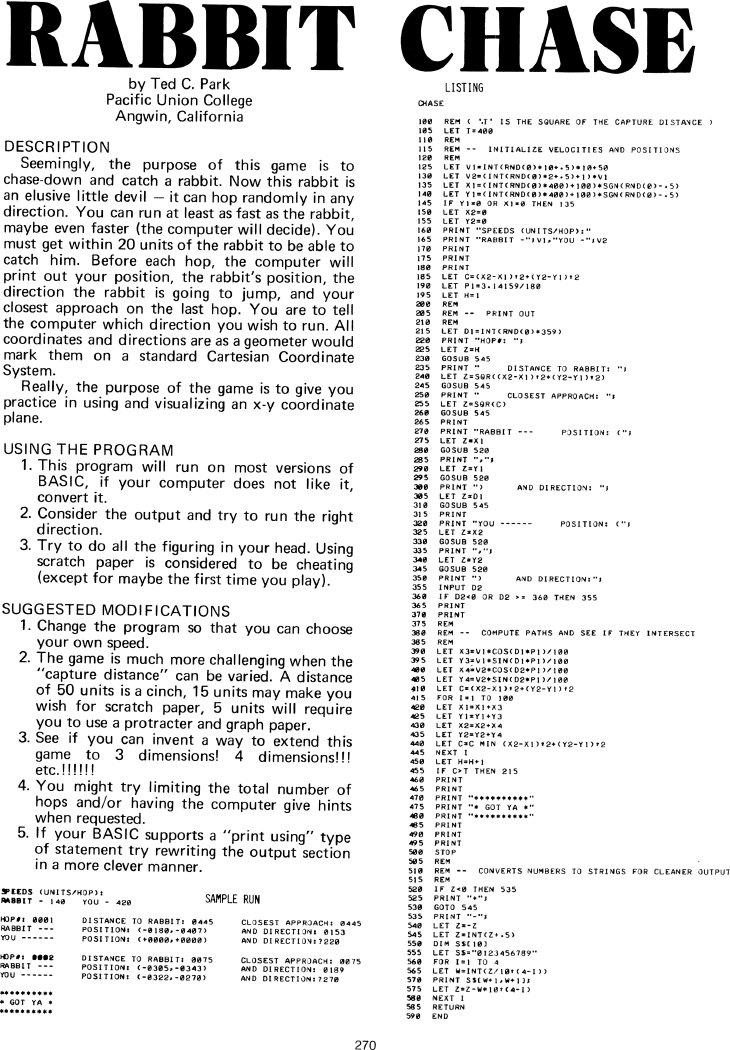The Best of Creative Computing Volume 1 (published 1976)

 `Page 270 << PREVIOUS >> NEXT Jump to page: Go to contents Go to thumbnails`

Rabbit Chase (using X-Y coordinate plane, BASIC computer game, BASIC program listing, sample run)```RABBIT
by Ted C.Park
Pacific Union College
Angwin, California
DESCRIPTION
Seemingly, the purpose of this game is to
chase-down and catch a rabbit. Now this rabbit is
an elusive little devil - it can hop randomly in any
direction. You can run at least as fast as the rabbit,
maybe even faster (the computer will decide). You
must get within 20 units of the rabbit to be able to
catch him. Before each hop, the computer will
print out your position, the rabbit's position, the
direction the rabbit is going to jump, and your
closest approach on the last hop. You are to tell
the computer which direction you wish to run. All
coordinates and directions are as a geometer would
mark them on a standard Cartesian Coordinate
System.

Really, the purpose of the game is to give you
practice in using and visualizing an x-y coordinate
plane.

USING THE PROGRAM
1. This program will run on most versions of
BASIC, if your computer does not like it,
convert it.

2. Consider the output and try to run the right
direction.

scratch paper is considered to be cheating
(except for maybe the first time you play).

SUGGESTED MODIFICATIONS
1. Change the program so that you can choose

2. The game is much more challenging when the
"capture distance" can be varied. A distance
of 50 units is a cinch, 15 units may make you
wish for scratch paper, 5 units will require
you to use a protracter and graph paper.

3. See if you can invent a way to extend this
game to 3 dimensions! 4 dimensions!!!

etc.!!!!!!

4. You might try limiting the total number of
hops and/or having the computer give hints
when requested.

5. lf your BASIC supports a "print using" type
of statement try rewriting the output section
in a more clever manner.

SEEDS (UNITS/HOPH):

RABBIT - 140 YOU - 420 SAMPLE RUN
HOP#: 0001 DISTANCE TO RABBIT: 0445 CLOSEST APPROACH: 0445
RABBIT --- POSITION: (-0I80,-0407) AND DIRECTION! 0153
YOU ---- -- POSITION: (+0000,*0000) AND DIRECTION:220
HOP#: 0002 DISTANCE T0 RABBIT: 0075 CLOSEST APPROACH: 0075
RABBIT---  POSITION: (-0305,-0343) AND DIRECTION: 0189
YOU------  POSITION: (-0322,-0270) AND DIRECTION:?270
**********
* GOT YA *
**********
LISTING
CHASE
100 REM (",T" IS THE SQUARE OF THE CAPTURE DISTANCE)
105 LET T=400
110 REM
115 REM --INITIALIZE VELOCITIES AND POSITION
120 REM
125 LET V1=INT(RND(0)*10*.5)*10+50
130 LET V2=INT(RND(0)*2*.5)+1)*V1
135 LET X1=INT(RND(0)*400)+100*SGN(RND(0)-.5)
140 LET Y1=INT(RND(0)*400)+100*SGN(RND(0)-.5)
145 LET X2=0
150 LET Y2=0
155 PRINT "SPEEDS (UNITS/HOP):"

160 PRINT "RABBIT -",V1,"YOU-",V2
165 PRINT
170 PRINT
175 PRINT
180 PRINT
185 LET C=(X2-X1)+2+(Y2-Y1)*2
190 LET P1=3*14159/180
195 LET H=1
200 REM
205 REM--PRINT OUT
210 REM
215 LED D1=INT(RND<0>*359)
220 PRINT "HOP#:";
225 LET Z=H
230 GOSUB 545
235 PRINT " DISTANCE TO RABBIT:";
240 LED Z=SQR((X2-X1)+2+(Y2-Y1)+2)
245 GOSUB 545
250 PRINT CLOSET APPROACH:";
255 LET Z=SQR(C)
260 GOSUB 545
265 PRINT
270 PRINT "RABBIT--- POSITION (";
275 LET Z=X1
280 GOSUB 520
285 PRINT ",";
290 LET Z=Y1
295 GOSUB 520
300 PRINT ") AND DIRECTION: ";
305 LET Z=D1
310 GOSUB 545
315 PRINT
320 PRINT "YOU ------ POSITION:(";
325 LET Z=X2
330 GOSUB 520
335 PRINT ",";
340 LET Z=Y2
345 GOSUB 520
350 PRINT "

355 INPUT 02
360 IF D2<0 OR D2>=360 THEN 355
365 PRINT
370 PRINT
375 REM
380 REM
385 REM
390 LET X3=V1*COS(D1*P1)/100
395 LET Y3=V1*SIN(D1*P1)/100
400 LET X4=V2*COS(D2*P1)/100
405 LET Y4=V2*SIN(D1*P1)/100
410 LET C=(X2-X1)*2+(Y2-Y1)*2
415 FOR I=1 TO 100
420 LET X1=X1+X3
425 LET Y1=Y1+Y3
430 LET X2=X2+X4
435 LET Y2=Y2+Y4
440 LET C=C MIN (X2-X1)*2+(Y2-Y1)*2
445 NEXT I
450 LET H=H+1
455 IF C>T THEN 215
460 PRINT
465 PRINT
470 PRINT "**********"

475 PRINT "* GOT YA *"

480 PRINT "**********"

485 PRINT
490 PRINT
495 PRINT
500 STOP
505 REM
510 REM -- CONVERTS NUMBERS TO STRINGS FOR CLEANER OUTPUT
515 REM
520 IF Z<0 THEN 535
525 PRINT "+";
530 GOTO 545
535 PRINT "-";
540 LET Z=-Z
545 LET Z=INT(Z+.5)
550 DIM S\$(10)
555 LET S\$="0123456789"

560 FOR I= 1 TO 4
565 LET W=INT(Z/10*)(4-I))
570 PRINT S\$(W+1,W+I);
575 LET Z=Z-W*10+(4-I)
580 NEXT I
585 RETURN
590 ENDPage 270        << PREVIOUS        >> NEXT        Jump to page:                Go to contents        Go to thumbnails

<!--